# GATE Solved Paper 2017-19 - GATE 2018

• A

0, c• B

0, a + 2• C

‘0’, ‘a + 2’• D

‘0’, ‘c’• Option : A
• Explanation :
So ‘a’ + 2 will be ‘c’, so Our next node p = {‘1’, ‘0’, ‘c’} and output will be 0, c. So answer is C

• Option : A
• Explanation :
E1 entries > E2 entities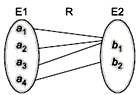So Every entity in E1 is associated with exactly one entity in E2. So options A is correct options.

• Option : D
• Explanation : The Given functions is, an = 2n + 3
generating function for 1 is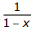and n is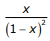, the generating function for an is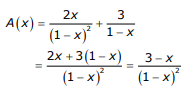Which is option (D).

• A

P ⊕ Q = P ⊙ Q• B

P ⊕ Q = P ⊙ Q• C

PQ = P ⊕ Q• D

(P ⊕ P) ⊕ Q ≠ (P ⊙P) ⊕ Q• Option : D
• Explanation :
(P ⊕ P) ⊕ Q = (P ⊙P) ⊕ Q false
(P ⊕ P) ⊕ Q = 1 ⊕ Q = Q
(P ⊙ P) ⊕ Q = 0 ⊕ Q = Q
(P ⊕ P) ⊕ Q ≠ (P ⊙ P) ⊕ Q

• A

Only I and II are necessarily true• B

Only II is necessarily true• C

Only I and III are necessarily true• D

Only II and III are necessarily true• Option : D
• Explanation : Only Eigen vector is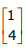multiples means that eigen value is repeated since if eigen values were distinct we will get one more independent eigen vector. So, II P has repeated eigen value is true. I need not be true since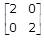has repeated eigen values and yet it is invertible. III is true since if matrix has repeated eigen values then it cannot be diagonalizable.
Related Quiz.
GATE 2018